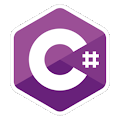Programming Course in C# ¡Free!

# Many numbers and sum

Proposed exercise

Create a program which asks the user for several numbers (until he enters "end" and displays their sum). When the execution is going to end, it must display all the numbers entered, and the sum again, as follows:

Enter a number: 5
Sum = 5
Enter a number: 3
Sum = 8
Enter a number: end
The numbers are: 5 3
The sum is: 8

Output

Solution

```using System;
public class ManyNumbers
{
public static void Main()
{
float[] numbers = new float;
float total = 0.0f;

int countArray = 0;